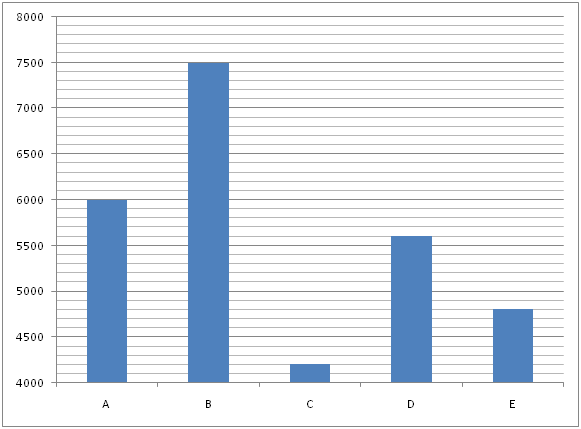# LIC AAO/SBI PO Prelims Quantitative Aptitude Questions 2019 (Day-07)

Dear Aspirants, Our IBPS Guide team is providing new series of Quantitative Aptitude Questions for LIC AAO/SBI PO 2019 so the aspirants can practice it on a daily basis. These questions are framed by our skilled experts after understanding your needs thoroughly. Aspirants can practice these new series questions daily to familiarize with the exact exam pattern and make your preparation effective.

[WpProQuiz 5573]

### Click Here for SBI PO Pre 2019 High-Quality Mocks Exactly on SBI Standard

Directions (Q. 1 – 5): In the following questions, two equations I and II are given. You have to solve both the equations and give answer as,

a) If x > y

b) If x ≥ y

c) If x < y

d) If x ≤ y

e) If x = y or the relation cannot be established

1) I) x2 – 6x – 72 = 0

II) y2 + 16x – 57 = 0

2) I) 2x2 – 7x – 39 = 0

II) 3y2 – 5y – 28 = 0

3) I) 5x – 2y = 19

II) 3x – 5y = 0

4) I) x3 = (1612 ÷ 13) + 36 % of 1200 – 232

II) y2 = 9

5) I) 6x2 – 49x + 99 = 0

II) 5y2 + 17y + 14 = 0

Directions (Q. 6 – 10): Study the following information carefully and answer the given questions:

The following bar graph shows the cost price (In Rs.) of different types of items.6) Find the marked price of Item A, if the shopkeeper allows a discount of 20 % but he gains 10 %?

a) Rs. 8400

b) Rs. 8050

c) Rs. 8250

d) Rs. 8500

e) None of these

7) The shopkeeper allows two successive discounts of 20 % and 10 % while buying the Item C. If the mark-up percentage of Item C is 25 %, then find the selling price of Item C?

a) Rs. 3780

b) Rs. 3620

c) Rs. 3560

d) Rs. 3340

e) None of these

8) Find the average cost price of all the given items together?

a) Rs. 5580

b) Rs. 5340

c) Rs. 5760

d) Rs. 5620

e) None of these

9) Find the discount % on Item D, if the shopkeeper marked the Item D for Rs. 8750 and gain % is 25 %?

a) 15 %

b) 20 %

c) 10 %

d) 25 %

e) None of these

10) Find the difference between the selling price of Item B to that of Item E, if the shopkeeper gains while selling the Item B and Item E is Rs. 300 and Rs. 200 respectively?

a) Rs. 2700

b) Rs. 2650

c) Rs. 2800

d) Rs. 2950

e) None of these

Direction (1-5) :

I) x2 – 6x – 72 = 0

(x – 12) (x + 6) = 0

X = 12, – 6

II) y2 + 16x – 57 = 0

(y + 19) (y – 3) = 0

Y = – 19, 3

Can’t be determined

I) 2x2 – 7x – 39 = 0

2x2 + 6x – 13x – 39 = 0

2x (x + 3) – 13(x + 3) = 0

(2x – 13) (x + 3) = 0

X = 13/2, -3 = 6.5, -3

II) 3y2 – 5y – 28 = 0

3y2 – 12y + 7y – 28 = 0

3y (y – 4) + 7(y – 4) = 0

(3y + 7) (y – 4) = 0

Y = -7/3, 4 = -2.33, 4

Can’t be determined

5x – 2y = 19 —> (1)

3x – 5y = 0 —> (2)

By solving the equation (1) and (2), we get,

X = 5, y = 3

X > y

I) x3 = (1612 ÷ 13) + 36 % of 1200 – 232

X3 = (1612/13) + (36/100)*1200 – 529

X3 = 124 + 432 – 529

X3 = 27

X = 3

II) y2 = 9

Y = 3, -3

X ≥ y

I. 6x2 – 49x + 99 = 0

6x2 – 27x – 22x + 99 = 0

3x (2x – 9) – 11(2x – 9) = 0

(3x – 11) (2x – 9) = 0

X= 11/3, 9/2

II. 5y2 + 17y + 14 = 0

5y2 + 10y + 7y + 14 = 0

5y (y + 2) +7 (y + 2) = 0

(5y + 7) (y + 2) = 0

Y= -7/5, -2

X > y

Direction (6-10) :

Cost price of item A = Rs. 6000

MP*[(100 – Discount %)/100] = CP*[(100 + Gain %)/100]

MP*(80/100) = 6000*(110/100)

MP = 6000*(110/100)*(100/80)

MP = Rs. 8250

Cost price of Item C = Rs. 4200

Mark-up % = 25 %

Marked price = CP*[(100 + Mark-up %)/100]

= > 4200*(125/100) = Rs. 5250

Selling price = 5250*(80/100)*(90/100) = Rs. 3780

The average cost price of all the given items together

= > (6000 + 7500 + 4200 + 5600 + 4800)/5

= > 28100/5 = Rs. 5620

Cost price of item D = Rs. 5600

Marked price of Item D = Rs. 8750

MP*[(100 – Discount %)/100] = CP*[(100 + Gain %)/100]

8750*[(100 – D%)/100] = 5600*(125/100)

100 – D% = 5600*(125/100)*(100/8750)

100 – D% = 80

D% = 100 – 80 = 20 %

The selling price of Item B = 7500 + 300 = Rs. 7800

The selling price of Item E = 4800 + 200 = Rs. 5000

Required difference = 7800 – 5000 = Rs. 2800